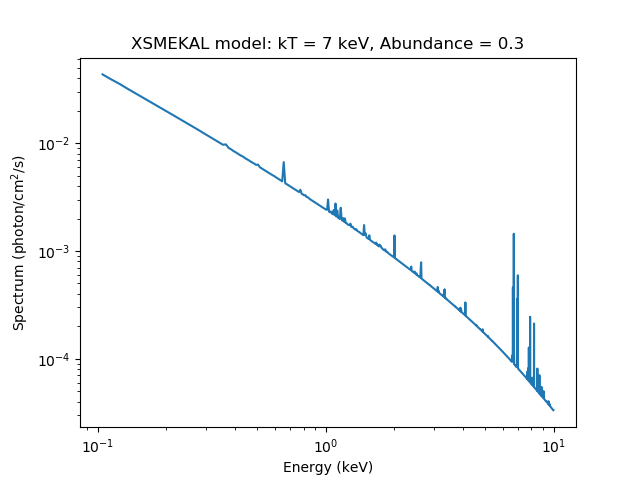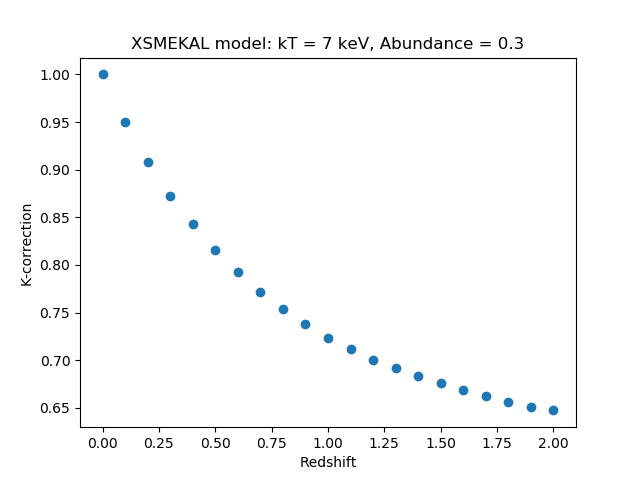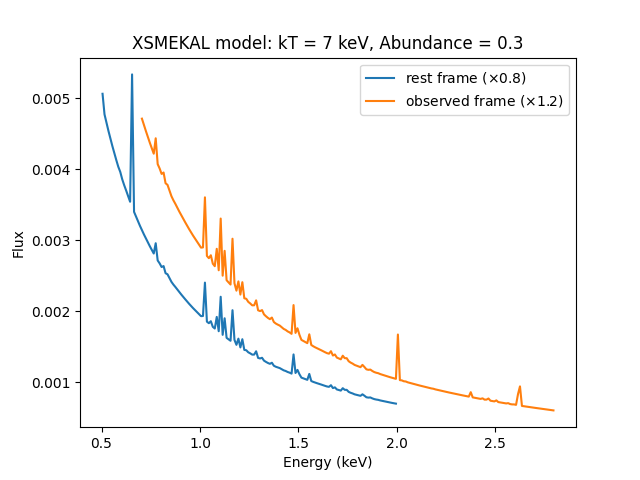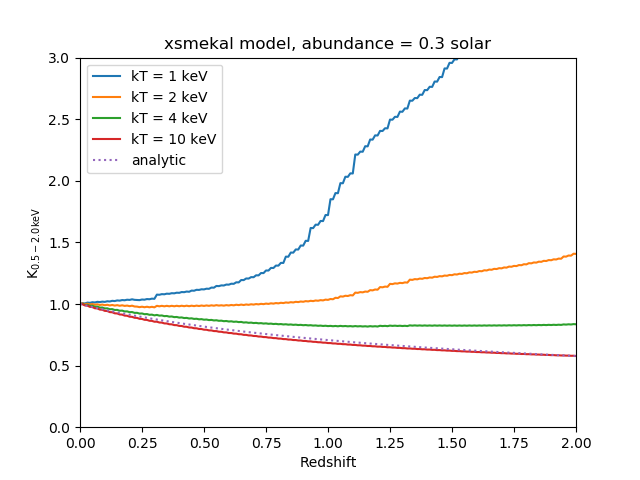# Calculating K-corrections using Sherpa## Overview

#### Synopsis:

In this thread we demonstrate the use of the Sherpa calc_kcorr function to calculate K-corrections for any of the spectral models available from Sherpa. As an example, we calculate the corrections necessary for the flux measured in the 0.5-2.0 keV energy range from a thermal plasma (as described by the Mewe-Kaastra-Liedahl—aka MEKAL—model) for a range of redshifts and gas temperatures. The aim is to emulate the figure presented in Appendix B of Jones et al. 1998, ApJ, 495, 100-114.

#### Purpose:

When observing extragalactic sources, the energies of the detected photons are $$(1+z)$$ times lower than the energy they had when they were emitted. This means that the flux—and hence luminosity—you measure within a certain passband on Chandra (or other satellite) does not match the same passband in the rest-frame of the object. In order to compare the luminosity of different objects, the values should be corrected to a common rest-frame passband; this correction is known as the "K-correction".

Last Update: 6 Dec 2022 - reviewed for CIAO 4.15, fixed a redundant linestyle argument that throws a warning by newer versions of matplotlib..

## Getting started: setting up the spectral model

In order to calculate the K-correction of a model in Sherpa, we simply define the model using the set_source command, assign it to a Sherpa dataset ID (even if we are not interested in fitting the model to a data spectrum), and then specify, with the calc_kcorr function, our desired redshift and energy range for this model.

In the following example, we use the Mewe-Kaastra-Liedahl thermal plasma model from the XSPEC library (xsmekal), setting the plasma temperature to 7 keV and the metal abundance to 0.3 times the cosmic abundance.

sherpa> set_source(xsmekal.plasma)
sherpa> plasma.kt = 7
sherpa> plasma.abundanc = 0.3


Since we are interested only in the ratio of the fluxes within two passbands, the value of the model normalization is not important; however, it is important that the redshift be left at the default (frozen) value of 0, and not changed to the value of the source of interest. Note that the Sherpa command show_source may be used to examine the model parameter settings, but not until the model has been assigned to a Sherpa data set ID, as shown in the next section.

## Evaluating the model

To calculate the K-correction, we need to access the model spectrum—i.e., the flux in units of ergs cm-2 s-1 for each energy bin.

Since we are interested in the unconvolved model spectrum, we do not need to establish an instrument response model (i.e., supply an ARF or RMF). While it is possible to use the calc_kcorr command with a convolved model, it will only work if the associated responses covers the required energy range—the observed range multiplied by $$(1.0+z_{\mathrm{max}})$$, which may not be the case if using broad energy bands, such as 2-10 keV.

### 1. Defining the energy grid of the model

Since we are not fitting the model to a source data spectrum, Sherpa does not know what energy grid over which to calculate the model. We therefore make use of the dataspace1d command to create a blank data set with the desired grid. It is important to ensure that the energy grid covers both the observed and rest-frame energy bands.

For the rest-frame energy range (0.5-2.0 keV) and redshift range (0-2) considered in this example, we need to ensure that the model is evaluated over at least 0.5 to 4.0 keV. It does not matter if the energy range is larger than that, so we choose 0.1 to 10 keV, with a grid spacing of 0.01 keV:

sherpa> dataspace1d(0.1, 10, 0.01)

sherpa> show_data()
Data Set: 1
Filter: 0.1000-10.0000 x
name      = dataspace1d
xlo       = Float64
xhi       = Float64
y         = Float64
staterror = None
syserror  = None


### 2. Displaying the model

Now that our model (with default model ID "1") is associated with a data set (data set ID "1")—the blank data set created with the dataspace1d command—we may use the show_source command to examine the model parameter settings:

sherpa> show_source()

Model: 1
xsmekal.plasma
Param        Type          Value          Min          Max      Units
-----        ----          -----          ---          ---      -----
plasma.kT    thawed            7       0.0808         79.9        keV
plasma.nH    frozen            1        1e-05        1e+19       cm-3
plasma.Abundanc frozen          0.3            0         1000
plasma.redshift frozen            0       -0.999           10
plasma.switch frozen            1            0            1
plasma.norm  thawed            1            0        1e+24


The Sherpa plot_source command may be used to display the unconvolved model amplitudes in photons cm-2 s-1, and the appropriate matplotlib commands for setting the axis labels and title of the resulting plot, as shown below (Figure 1):

sherpa> plot_source(xlog=True, ylog=True)

sherpa> plt.xlabel("Energy (keV)")
sherpa> plt.ylabel("Spectrum (photon/cm$^2$/s)")
sherpa> plt.title("XSMEKAL model: kT = 7 keV, Abundance = 0.3")


### Figure 1: Model Spectrum### Figure 1: Model Spectrum

Plot of the unconvolved Mewe-Kaastra-Liedahl thermal plasma model ("xsmekal") over the 0.1 to 10 keV energy range.

## Calculating the K-correction

Sherpa includes the calc_kcorr function for calculating the K-correction for a given spectral model, redshift, and pair of energy passbands. Below we show how to use this function, and then discuss how the function works.

### Using

Now that we have defined the source model spectrum, we can use the calc_kcorr function to calculate the K-correction. To calculate the K-correction for an object at redshift z=0.4, using the model assigned to (empty) data set 1, we would do:

sherpa> calc_kcorr(z=0.4, obslo=0.5, obshi=2.0, id=1)
0.8388622622766366


This command returns the K-correction for a flux measured in the observed 0.5-2.0 keV energy band, in order to get the flux in the rest-frame 0.5-2.0 keV band for an object at a redshift of 0.4. If you want to convert to a different rest-frame energy band, simply supply the band as extra parameters, as shown below for the rest-frame 0.1-2.4 keV energy range.

sherpa> calc_kcorr(z=0.4, obslo=0.5, obshi=2.0, restlo=0.1, resthi=2.4, id=1)
1.3543060109802925


Instead of a single (i.e. scalar) value for the redshift, a one-dimensional array can be supplied, containing a range of redshifts. Here we calculate the correction values for the redshift range z=0-2 with a step size of 0.1 (using the Numpy 'arange' function), and then plot K-correction versus redshift (Figure 2):

sherpa> z = np.arange(0.0, 2.1, 0.1)
sherpa> kc = calc_kcorr(z=z, obslo=0.5, obshi=2.0, id=1)

sherpa> plt.clf()
sherpa> plt.plot(z, kc, 'o')
sherpa> plt.xlabel("Redshift")
sherpa> plt.ylabel("K-correction")
sherpa> plt.title("XSMEKAL model: kT = 7 keV, Abundance = 0.3")


### Figure 2: K-correction between z=0 and 2### Figure 2: K-correction between z=0 and 2

Plot displaying the K-correction as a function of redshift.

### How calc_kcorr works

The K-correction is calculated as the ratio of the flux in the rest-frame energy band to that in the observed band (for instance, see Appendix B of Jones et al. 1998, ApJ, 495, 100-114). If we denote the low and high energies of the rest-frame band as $$E_{\mathrm{lo}}$$ and $$E_{\mathrm{hi}}$$, then for a source at redshift $$z$$, the flux within the observed band would be as follows:

1. observed band: $$e_{\mathrm{lo}}(1+z)$$ to $$e_{\mathrm{hi}}(1+z)$$
2. rest-frame band: $$E_{\mathrm{lo}}$$ to $$E_{\mathrm{hi}}$$

The Sherpa calc_energy_flux command is used to compute the unconvolved model energy flux within the desired energy range, in units of ergs cm-2 s-1:

sherpa> z = 0.4

sherpa> rest_flux = calc_energy_flux(0.5, 2., id=1)

sherpa> obs_flux  = calc_energy_flux(0.5*(1+z), 2.*(1+z), id=1)

sherpa> rest_flux
5.574282179843434e-10

sherpa> obs_flux
6.64505060069703e-10

sherpa> kcorr = rest_flux / obs_flux

sherpa> kcorr
0.8388622622766366


The calc_energy_flux() function returns the source flux within the rest-frame 0.5-2.0 keV range and the observed 0.7-2.8 (0.5×1.4 to 2.0×1.4) keV range; dividing the two values gives the K-correction for this spectral model, redshift, and energy range.

### Displaying the models being evaluated

Here we demonstrate how to use the Sherpa get_model_plot function to read the model amplitudes into Python variables and then display them with matplotlib plotting commands (type "get*?" at the Sherpa prompt for the full list of Sherpa get commands). We evaluate the model over both the observed and rest-frame 0.5-2.0 keV energy ranges by defining the appropriate energy grids with the dataspace1d command, and then plot the resulting models together in one window.

sherpa> # Define energy grids over which to evaluate the model:
sherpa> # rest-frame energy range
sherpa> dataspace1d(0.5, 2.0, 0.01, id=2)
sherpa> # observed energy range
sherpa> dataspace1d(0.7, 2.8, 0.01, id=3)

sherpa> Evaluate the model over the defined energy grids
sherpa> by assigning it to the blank data sets 2 and 3
sherpa> set_source(2, plasma)
sherpa> set_source(3, plasma)

sherpa> # Retrieve the model amplitudes:
sherpa> rest_dep = get_model_plot(2).y
sherpa> obs_dep  = get_model_plot(3).y

sherpa> print(rest)
sherpa> print(obs)

sherpa> # Retrieve the energy axis information
sherpa> rest_indep = get_model_plot(2).x
sherpa> obs_indep  = get_model_plot(3).x

sherpa> print(rest_indep)
sherpa> print(obs_indep)

sherpa> # Display the two energy ranges (with a slight offset in y):
sherpa> plt.clf()
sherpa> plt.plot(rest_indep, rest_dep*0.8, '-', label=r'rest frame ($\times 0.8$)')
sherpa> plt.plot(obs_indep, obs_dep*1.2, '-', label=r'observed frame ($\times 1.2$)')

sherpa> #Customize the plot:
sherpa> plt.legend()
sherpa> plt.xlabel("Energy (keV)")
sherpa> plt.ylabel("Flux")
sherpa> plt.title("XSMEKAL model: kT = 7 keV, Abundance = 0.3")


Figure 3 shows the resulting plot, with the models offset for display purposes.

### Figure 3: Model spectrum after filtering on energy### Figure 3: Model spectrum after filtering on energy

The two curves show the unconvolved model spectrum evaluated over the rest- and observed-frame energy ranges, respectively (offset from each other by 20 percent for clarity).

The model used here is the xsmekal plasma model with a temperature of 7 keV, metallicity abundance of 0.3 times the cosmic abundance, and a redshift of 0. The observed energy range is taken to be 0.5-2.0 keV and the source redshift (for calculating the rest-frame energy range) is z=0.4.

## Changing the model parameters

In this section we show how calc_kcorr can be used to generate K-corrections for a range of values of a given model parameter (here the plasma temperature). The aim is to emulate Figure 7 of Jones et al. 1998, ApJ, 495, 100-114. We therefore calculate the corrections for plasma temperatures of 1, 2, 4, and 10 keV using the following commands, which are available as a Sherpa Python script (plot_kcorrs.py):

#Clear existing data and model definitions in the current Sherpa session:
clean()

#Define the range of redshifts in which we are interested (here using
the Numpy 'arange' function):

z = np.arange(0.0, 2.01, 0.01)

#Define an energy grid which covers at least 0.5-2.0 keV for the
redshift range z = 0 to 2:

elo = 0.5
ehi = 2.0

dataspace1d(0.1, 7.0, 0.01, id=1)

#Define the model:

set_source(xsmekal.plasma)
plasma.abundanc = 0.3

#Calculate the K-correction for a 1 keV plasma model:
plasma.kt = 1
k1 = calc_kcorr(z, elo, ehi, id=1)

#Calculate the K-correction for a 2 keV plasma model:
plasma.kt = 2
k2 = calc_kcorr(z, elo, ehi, id=1)

#Calculate the K-correction for a 4 keV plasma model:
plasma.kt = 4
k4 = calc_kcorr(z, elo, ehi, id=1)

#Calculate the K-correction for a 10 keV plasma model:
plasma.kt = 10
k10 = calc_kcorr(z, elo, ehi, id=1)

#Calculate the analytic solution using a power law
#(using the numpy Python package for mathematical operations on arrays):
z = np.array(z)
kpl = 1./np.sqrt(1+z)

#Plot the model curves:

from matplotlib import pyplot as plt

plt.figure()

plt.plot(z, k1, '-', label='kT = 1 keV')
plt.plot(z, k2, '-', label='kT = 2 keV')
plt.plot(z, k4, '-', label='kT = 4 keV')
plt.plot(z, k10, '-', label='kT = 10 keV')

plt.plot(z, kpl, linestyle='dotted', label='analytic')

plt.xlabel("Redshift")
plt.ylabel(r"K$_{0.5-2.0 {\rm keV}}$")
plt.title("xsmekal model, abundance = 0.3 solar")

plt.xlim(0, 2)
plt.ylim(0, 3)

plt.legend()

plt.savefig("plasmakT_kcorr.png")
save("sherpa_plot_session.save")

#finished
quit()


Figure 4 shows the resulting plot, which can be compared to Figure 7 of Jones et al. (1998).

### Figure 4: Corrections for a family of models### Figure 4: Corrections for a family of models

The models were chosen to be the same as used in Appendix B (Figure 7) of Jones et al. 1998, ApJ, 495, 100-114.

## Caveats

### 1. Accuracy

The accuracy of the K-correction is determined by the width of the energy bins. We shall illustrate this by using the xspowerlaw model, for which an analytic solution for the K-correction exists: for a photon index of $$\Gamma$$, the K-correction is given by $$k(z) = (1+z)^{\Gamma-2}$$. Below we calculate the corrections for two bin widths—0.1 and 0.01 keV—and compare them to the analytic solution.

#Clear existing data and model definitions in the current Sherpa session:
sherpa> clean()

# Define a power law model:
sherpa> set_source(xspowerlaw.p1)
sherpa> p1.phoindex = 3

#Define the redshift range and the analytic solutions:
sherpa> z = [0, 0.2, 0.4, 0.6, 0.8, 1]
sherpa> z = np.array(z)
sherpa> kz = np.power((1+z),(3-2))

#Calculate the K-correction for the model evaluated over two different energy grids:
sherpa> dataspace1d(0.1, 10, 0.1, id=1)
sherpa> kz1 = calc_kcorr(z, 0.5, 2.0, id=1)

sherpa> set_source(2, p1)
sherpa> dataspace1d(0.1, 10, 0.01, id=2)
sherpa> kz2 = calc_kcorr(z, 0.5, 2.0, id=2)

#Write the results to file with the write_arrays routine available in the
crates_contrib module of the CIAO contributed scripts package.

(Note that the Sherpa command save_arrays may also be used,
however it does not allow the user to specify the Python string format in which the
data values should be written to file, as write_arrays does).

sherpa> from crates_contrib.utils import *

sherpa> write_arrays("out.dat", [z, kz, kz1, kz2], format='{:.5g}')

sherpa> !more out.dat
0 1 1 1
0.2 1.2 1.2046 1.2003
0.4 1.4 1.409 1.4007
0.6 1.6 1.6133 1.601
0.8 1.8 1.8176 1.8014
1 2 2.0218 2.0017



The output columns list the redshift, analytic solution, then the values calculated by calc_kcorr for the two bin widths (0.1 keV and then 0.01 keV). As can be seen, the smaller grid spacing produces results closer to the true value. The choice of grid spacing depends on the model(s) used and the desired accuracy: for instance, in this example the error introduced by using a bin width of 0.1 keV is likely to be much smaller than the error on the measured flux of the source or uncertainties in the model parameters used to calculate the K-correction.

## Scripting It

The file fit.py is a Python script which performs the primary commands used above; it can be executed by typing %run fit.py on the Sherpa command line.

The Sherpa script command may be used to save everything typed on the command line in a Sherpa session:

sherpa> script(filename="sherpa.log", clobber=False)


(Note that restoring a Sherpa session from such a file could be problematic since it may include syntax errors, unwanted fitting trials, et cetera.)

## History

 14 Dec 2004 updated for CIAO 3.2: script version and path 21 Dec 2005 reviewed for CIAO 3.3: no changes 01 Dec 2006 reviewed for CIAO 3.4: no changes 16 Aug 2010 Sherpa commands and ChIPS plots updated for CIAO 4.2 05 Dec 2013 updated for CIAO 4.6: plots and calculated results updated, updated Python syntax. 13 Jan 2015 updated for CIAO 4.7: no content change, typos fixed. 11 Dec 2015 reviewed for CIAO 4.8: no content change. 02 Nov 2016 reviewed for CIAO 4.9: no content change, updated outputs. 31 May 2018 reviewed for CIAO 4.10: no content change. 11 Dec 2018 reviewed for CIAO 4.11, no content change, equations now use $$\LaTeX$$. 09 Dec 2019 Updated plots to use Matplotlib rather than ChIPS commands for CIAO 4.12. 31 Mar 2022 reviewed for CIAO 4.14, updated output and fixed typesetting. 06 Dec 2022 reviewed for CIAO 4.15, fixed a redundant linestyle argument that throws a warning by newer versions of matplotlib..